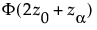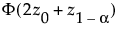• p* is the proportion of bootstrap samples with an estimate of the statistic of interest that is less than or equal to the original estimate.
 • z0 is the p* quantile of a standard normal distribution.
 • zα is the α quantile of a standard normal distribution.
The endpoints of a (1 - α) bias-corrected confidence intervals are given by quantiles of the bootstrap distribution:
 • The lower endpoint is thequantile.
 • The upper endpoint is thequantile.

Help created on 9/19/2017